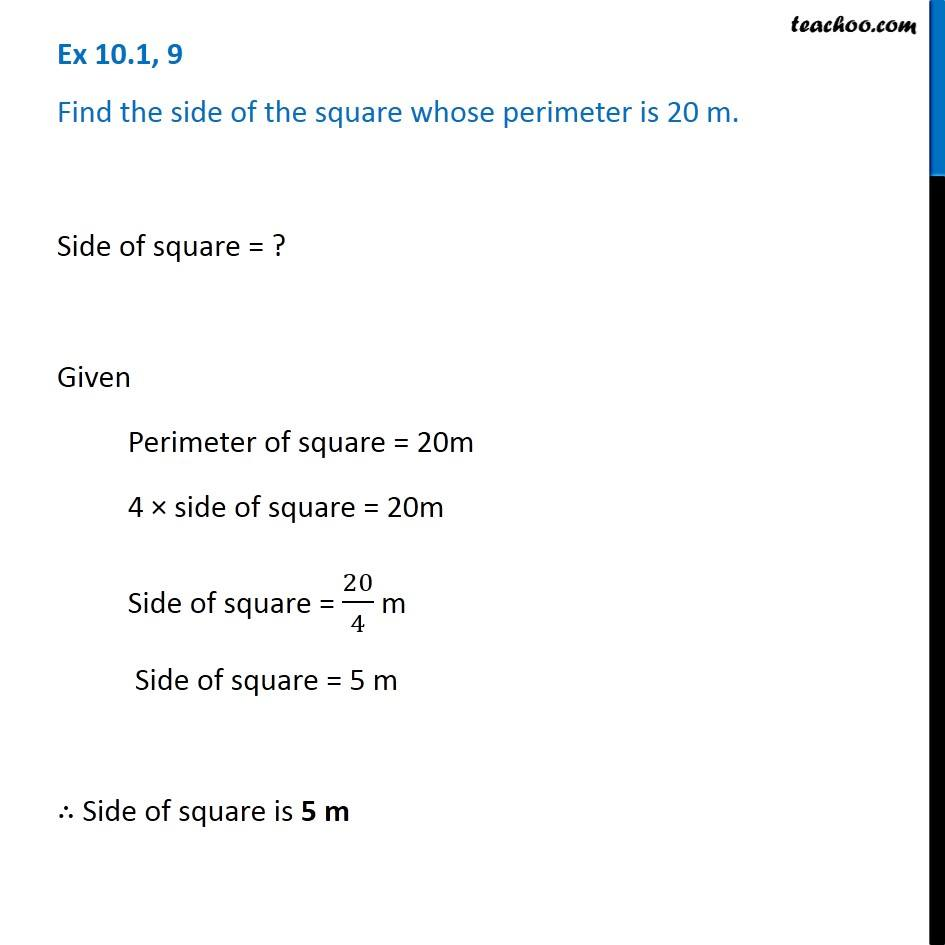Ex 10.1

Chapter 10 Class 6 Mensuration
Serial order wiseGet live Maths 1-on-1 Classs - Class 6 to 12

### Transcript

Ex 10.1, 9 Find the side of the square whose perimeter is 20 m. Side of square = ? Given Perimeter of square = 20m 4 × side of square = 20m Side of square = 20/4 m Side of square = 5 m ∴ Side of square is 5 m